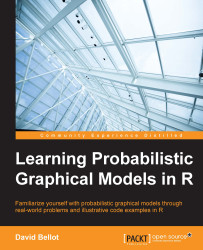•#### Learning Probabilistic Graphical Models in R#### Overview of this book

Probabilistic graphical models (PGM, also known as graphical models) are a marriage between probability theory and graph theory. Generally, PGMs use a graph-based representation. Two branches of graphical representations of distributions are commonly used, namely Bayesian networks and Markov networks. R has many packages to implement graphical models. We’ll start by showing you how to transform a classical statistical model into a modern PGM and then look at how to do exact inference in graphical models. Proceeding, we’ll introduce you to many modern R packages that will help you to perform inference on the models. We will then run a Bayesian linear regression and you’ll see the advantage of going probabilistic when you want to do prediction. Next, you’ll master using R packages and implementing its techniques. Finally, you’ll be presented with machine learning applications that have a direct impact in many fields. Here, we’ll cover clustering and the discovery of hidden information in big data, as well as two important methods, PCA and ICA, to reduce the size of big problems.
Learning Probabilistic Graphical Models in RCreditswww.PacktPub.comPrefaceFree Chapter
Probabilistic ReasoningExact InferenceLearning ParametersBayesian Modeling – Basic ModelsApproximate InferenceBayesian Modeling – Linear ModelsProbabilistic Mixture ModelsAppendixIndex## Chapter 7. Probabilistic Mixture Models

We have seen an initial example of mixture models, namely the Gaussian mixture model, in which we had a finite number of Gaussians to represent a dataset. In this chapter, we will focus on more advanced examples of mixture models, going again from the Gaussian mixture model to the Latent Dirichlet Allocation. The reason for so many models is that we want to capture various aspects of the data that are not easily captured by a mixture of Gaussian.

In many cases, we will use the EM algorithm to find the parameters of the model from the data. Also, it appears that most of the mixture models can have intractable solutions and need solutions on approximate inferences.

The first type of model we will see is a mixture of simple distributions. The simple distribution can be a Gaussian, a Bernoulli, a Poisson, and so on. The principle is always the same but the applications are different. If Gaussian distributions are nice for capturing clouds of points, Bernoulli...